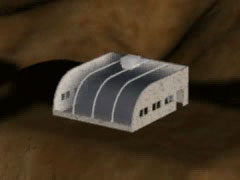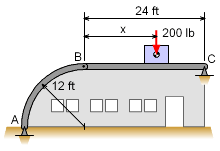Ch 5. Rigid Body Equilibrium Multimedia Engineering Statics 2-D and3-D Supports Equilibriumin 2-D Equilibriumin 3-D Indeterminate Objects 2 and 3 Force Members
 Chapter 1. Basics 2. Vectors 3. Forces 4. Moments 5. Rigid Bodies 6. Structures 7. Centroids/Inertia 8. Internal Loads 9. Friction 10. Work & Energy Appendix Basic Math Units Sections Search eBooks Dynamics Fluids Math Mechanics Statics Thermodynamics Author(s): Kurt Gramoll ©Kurt GramollSTATICS - CASE STUDY IntroductionHouse StructureProblem Diagram An architect has designed a greenhouse with a flat glass roof and a curved glass wall. Each section of the frame for this structure is two pieces. Where should the air conditioning unit be placed so that its weight is evenly distributed? What is known: The entire weight of the air conditioner is resting on one section of the frame. The section of frame consists of a quarter-circular arch with a radius of 12 ft, and a beam with a length of 24 ft. The arch is connected to the beam with a pin. The frame is supported at points A and C with pin supports. The air conditioning unit weighs 200 lb, and is resting on the beam. Question Where should the air conditioner be placed so that pins A, B, and C all have the same force magnitude acting at their respective joints? Approach Knowing that the frame is composed of a two-force and a three-force member, determine the geometry of the support reactions at A, B, and C. Use equilibrium equations to find equations for the reaction forces at B and C. Use geometry and the equations for the reaction forces at B and C to determine the position of the air conditioner which produces equal reaction force magnitudes at A, B, and C.

Practice Homework and Test problems now available in the 'Eng Statics' mobile app
Includes over 500 problems with complete detailed solutions.
Available now at the Google Play Store and Apple App Store.